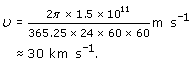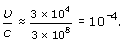The Big Bang

Start this free course now. Just create an account and sign in. Enrol and complete the course for a free statement of participation or digital badge if available.

Free course

# 6.2.3 Measurement of the angular distribution of the 3 K radiation

How are such angular distributions to be measured? One way, of course, is to take a radio telescope and swing it round the sky, taking readings in different directions. But as is clear from Figure 20(a), the atmosphere itself emits microwaves. There is therefore a grave danger, with this method, of picking up different contributions of atmospheric emission from different directions – more as you point your telescope close to the ground, with a long path through the atmosphere, less when it is vertical with the minimum path through the atmosphere. A better, and indeed simpler, way with a ground-based telescope is to leave the telescope in a fixed direction with respect to the Earth, say vertical, and let the Earth's rotation carry it round. In 24 hours, the telescope will be carried round a whole circle, allowing the measured intensity to be plotted against angle of observation as indicated in Figure 27.

Thus a practical prescription for looking for the translational motion of the Earth, relative to the frame in which the 3 K radiation is isotropic, is to look for a variation with a 24-hour period in the radiation detected by a fixed radio telescope. Such a search, for a variation of known period, can be carried out very sensitively. That is why we have looked at the data in two stages, first asking if the data look isotropic, and now asking more specifically for the magnitude of any 24-hour variation.

A further nice experimental feature is that the component being sought is of period 24 sidereal hours. Human interference, on the other hand, will tend to have a period of 24 solar hours. This small distinction (explained below) often allows a radio astronomer to sort out the pure astronomical signal from the strong terrestrial noise.

Sidereal Day

Looking at the Earth from outside the Solar System, one sees it revolving 366¼ times a year, not 365¼. From our position on the Earth, we see only 365¼ days, because one turn is taken up in the revolution round the Sun. A sidereal day is (1/366¼) of a year and a solar day is (1/365¼) of a year.

## Question 14

How large a change in apparent temperature of the 3 K radiation is expected due to the Earth's motion round the Sun? How large is it due to the Solar System's motion of 230 km s−1 round the Galaxy? (The data sheet gives information you may need to answer this question.)

The mean speed of the Earth round the Sun can be obtained by taking the orbit as a circle of radius 1.5 × 1011 m. The speedis then(Strictly, the orbit is not quite circular, so the speed varies somewhat above and below this value.)

From Equation 12 in Section 6.2, the maximum change in apparent temperature, ΔT, which this motion can cause at low frequencies arises when cosθ = 1. ΔT/T is then a fractionThe speed of the Solar System round the Galaxy about 230 km s−1, gives a ΔT/T of about (2.3 × 105)/(3 × 108) ≈ 10−3.

S357_1